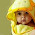## Best Method to Blink an LED using Arduino ?

by realfinetime  |  in LED at  03:24

First experiment that all the arduino beginners want to do is to blink an LED using Arduino. Blinking an LED using Arduino is very simple. Normally beginners use Arduino Uno or Arduino duemilanove. I used Arduino Mega here. Complete the circuit as shown in following diagram.

Connect an LED to the 13th digital pin of Arduino board through a current limiting series resistor. Value of current limiting resistance depends on your LED. I used 1 K resistance in my circuit. The cathode end of the LED must be connected to the Gnd pin of the Arduino board. Now upload the following program to your Arduino board. ( These procedures are same for all Arduino boards )

/*
Turns on an LED on for one second, then off for one second, repeatedly.

This example code is in the public domain.
*/

// Pin 13 has an LED connected on most Arduino boards.
// give it a name:
int led = 13;

// the setup routine runs once when you press reset:
void setup() {
// initialize the digital pin as an output.
pinMode(led, OUTPUT);
}

// the loop routine runs over and over again forever:
void loop() {
digitalWrite(led, HIGH);   // turn the LED on (HIGH is the voltage level)
delay(1000);               // wait for a second
digitalWrite(led, LOW);    // turn the LED off by making the voltage LOW
delay(1000);               // wait for a second
}

1.